2x 3x formula - Finding value

Chapter 3 Class 11 Trigonometric Functions
Concept wise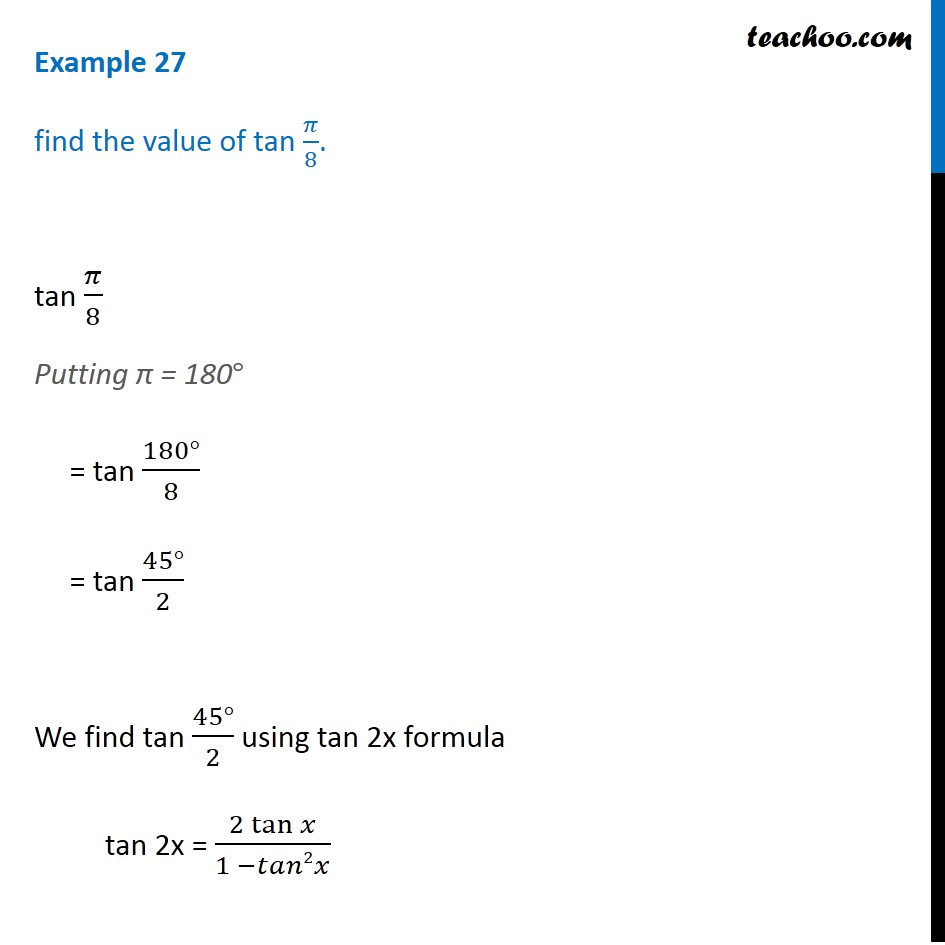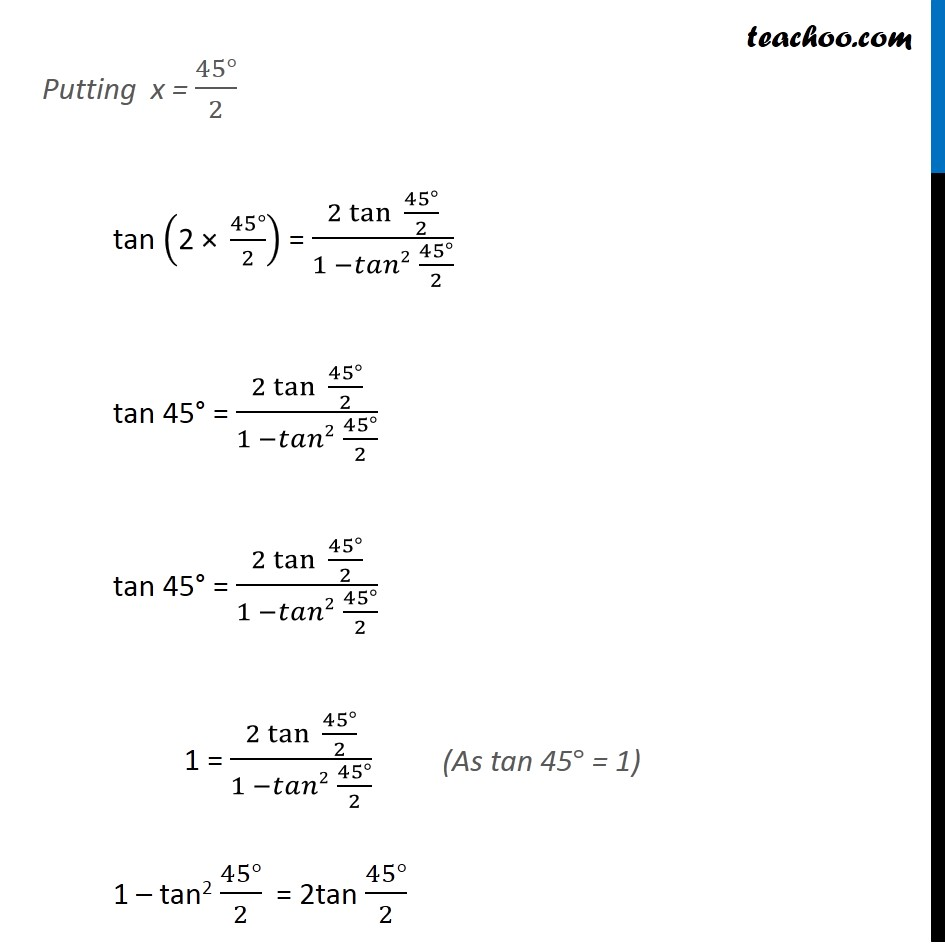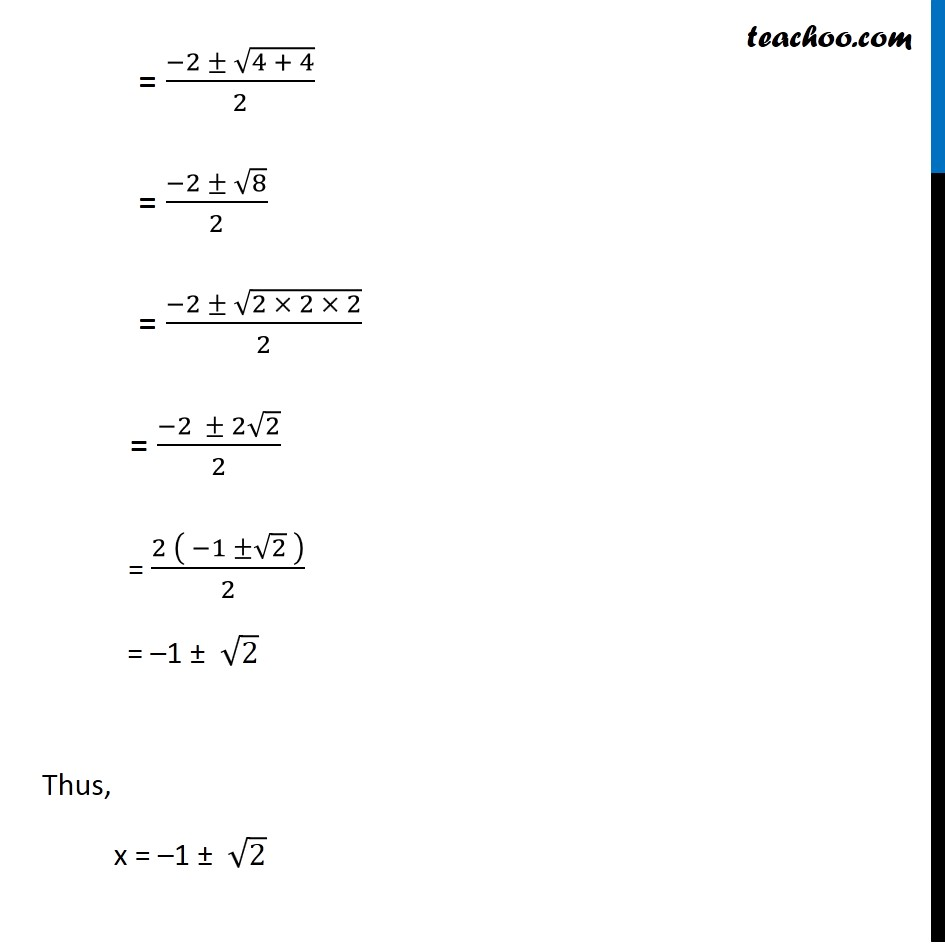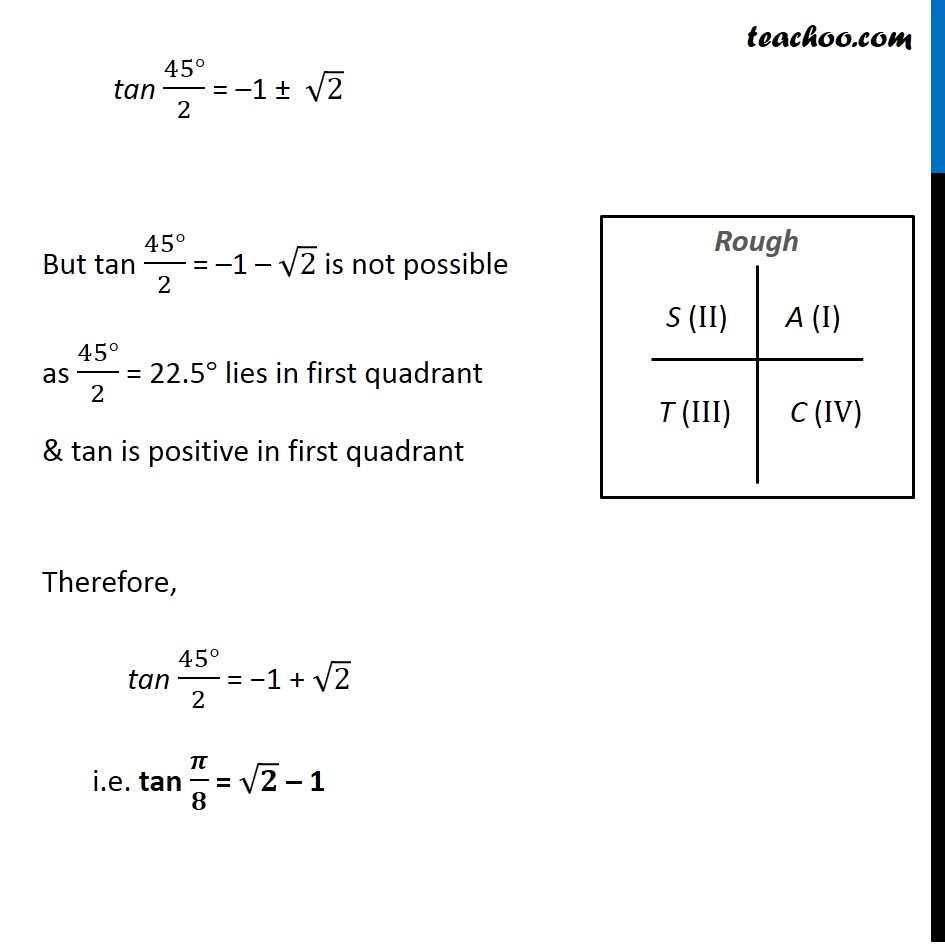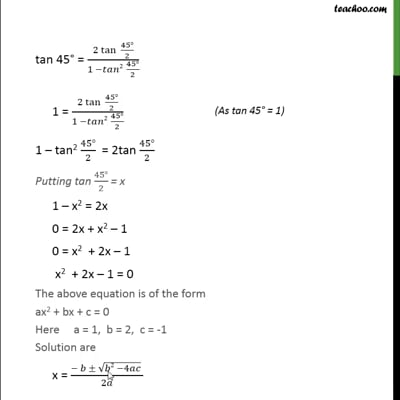This video is only available for Teachoo black users

Introducing your new favourite teacher - Teachoo Black, at only ₹83 per month

### Transcript

Example 27 find the value of tan 𝜋/8. tan 𝜋/8 Putting π = 180° = tan (180°)/8 = tan (45°)/2 We find tan (45°)/2 using tan 2x formula tan 2x = (2 tan⁡𝑥)/(1 −𝑡𝑎𝑛2𝑥) Putting x = (45°)/2 tan ("2 × " (45°)/2) = (2 tan⁡〖 (45°)/2〗)/(1 −𝑡𝑎𝑛2 (45°)/2) tan 45° = (2 tan⁡〖 (45°)/2〗)/(1 −𝑡𝑎𝑛2 (45°)/2) tan 45° = (2 tan⁡〖 (45°)/2〗)/(1 −𝑡𝑎𝑛2 (45°)/2) 1 = (2 tan⁡〖 (45°)/2〗)/(1 −𝑡𝑎𝑛2 (45°)/2) 1 – tan2 (45°)/2 = 2tan (45°)/2 (As tan 45° = 1) Let tan (𝟒𝟓°)/𝟐 = x So, our equation becomes 1 – x2 = 2x 0 = 2x + x2 – 1 x2 + 2x – 1 = 0 The above equation is of the form ax2 + bx + c = 0 where a = 1, b = 2, c = −1 Solution are x = (− 𝑏 ± √(𝑏2 −4𝑎𝑐) )/2𝑎 = (− 2 ± √((−2)2 − 4 × 1 × (−1)) )/(2 × 1) = (−2 ± √(4 + 4))/2 = (−2 ± √8)/2 = (−2 ± √(2 × 2 × 2))/2 = (−2 ± 2√2)/2 = (2 ( −1 ±√2 ))/2 = –1 ± √2 Thus, x = –1 ± √2 tan (45°)/2 = –1 ± √2 But tan (45°)/2 = –1 – √2 is not possible as (45°)/2 = 22.5° lies in first quadrant & tan is positive in first quadrant Therefore, tan (45°)/2 = −1 + √2 i.e. tan 𝝅/𝟖 = √𝟐 – 1